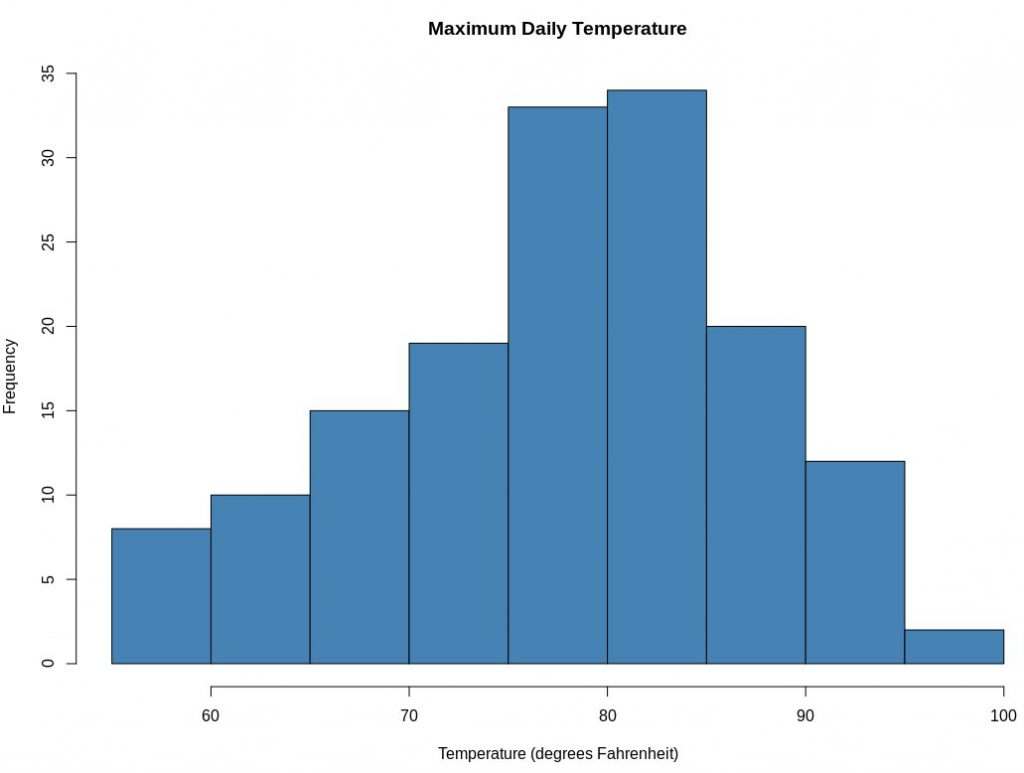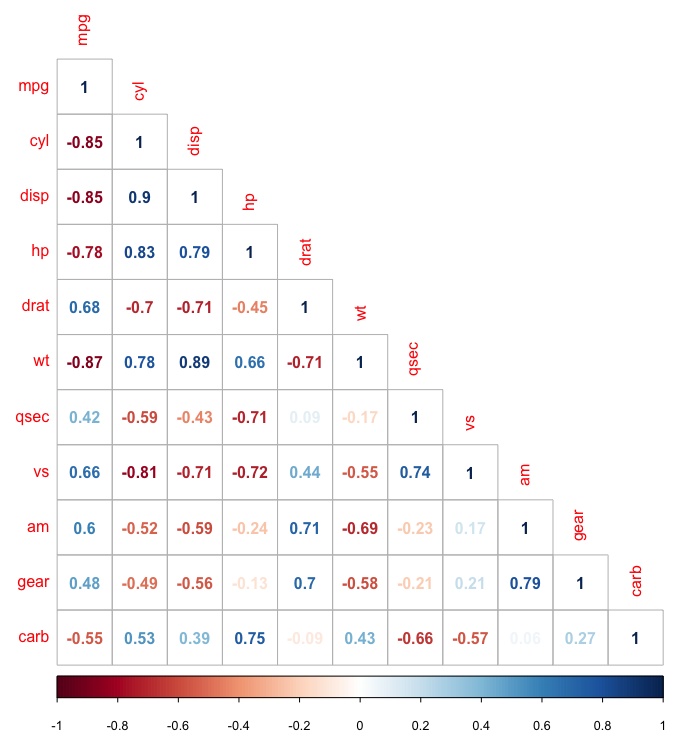HomeBlogData ScienceData Visualization in R programming: Top Visualizations For Beginners To Learn

# Data Visualization in R programming: Top Visualizations For Beginners To Learn

Last updated:
22nd Jan, 2020
Views
8 MinsView AllAnyone involved in Data Analysis has undoubtedly heard of and even dealt with Data Visualization. If you are a newbie, learn all about data visualization here. Data Visualization is a crucial part of Data Analysis and refers to the visual representation of data in the form of a graph, or chart, or bar, or any other format. Essentially, the purpose of Data Visualization is to represent or depict the relationship between the data and images.

The rise of Big Data has made it mandatory for Data Scientists and Data Analysts to simplify the insights obtained via visual representations for ease of understanding. Since Data Scientists and Analysts now work with large amounts of complex and voluminous datasets, Data Visualization has become more pivotal than ever. Data Visualization offers a visual or pictorial summary of the data at hand, thereby making it easier for Data Science and Big Data professionals to identify the hidden patterns and trends within the data.

Thanks to Data Visualization, professionals in the Data Science and Big Data fields need not browse extensively through thousands of rows and columns in a spreadsheet – they can refer to the visualization to understand where all the relevant information lies within a dataset.

Although we have numerous standalone and nifty Data Visualization tools like Tableau, QlikView, and d3.js, today, we are going to talk about Data Visualization in R programming language. R is an excellent tool for Data Visualization since it comes with many inbuilt functions and libraries that cover almost all Data Visualization needs.

In this post, we will discuss 8 R Data Visualization tools used by Data Scientists and Analysts the world over!

## Top 8 Data Visualization Tools

### 1. Bar Chart

Everyone is familiar with the bar charts that were taught in schools and colleges. In R Data Visualization with a bar chart, the concept and aim remain the same – it is to show a comparison between two or more variables. Bar charts depict the comparison between the cumulative total across various groups. The standard syntax to create a bar-chart in R is:

barplot(H,xlab,ylab,main, names.arg,col)

There are many different types of bar charts that serve unique purposes. While horizontal and vertical bar charts are the standard formats, R can create both horizontal and vertical bars in a chart. Besides, R also offers a stacked bar chart that lets you introduce different variables to each category. In R, the barplot() is used to create bar charts.

### 2. Histogram

Histograms work best with precise or numbers in R. This representation breaks the data into bins (breaks) and depicts the frequency distribution of these bins. You can tweak the bins and see what effect it has on the visualization pattern. The standard syntax for creating a histogram using R is:

hist(v,main,xlab,xlim,ylim,breaks,col,border)

Histograms provide a probability estimate of a variable, that is, the time period before the completion of a project. Each bar in a histogram represents the height of the number of values present in that range. The R language uses the hist() function for creating histograms.Source

### 3. Box Plot

A Box plot depicts five statistically significant numbers including the minimum, the 25th percentile, the median, the 75th percentile, and the maximum. Although a box plot shares many similarities with a bar chart, a box plot provides visualization for categorical and continuous variable data, instead of focusing only on categorical data. The standard syntax to create a boxplot in R is:

boxplot(x, data, notch, varwidth, names, main)

R creates box plots using the boxplot() function. This function can take in any number of numeric vectors, and draw a boxplot for each vector. Box plots are best-suited for visualizing the spread of the data and accordingly derive inferences based on it.

Learn data science courses from the World’s top Universities. Earn Executive PG Programs, Advanced Certificate Programs, or Masters Programs to fast-track your career.

upGrad’s Exclusive Data Science Webinar for you –

Watch our Webinar on The Future of Consumer Data in an Open Data Economy

## Explore our Popular Data Science Courses

 Executive Post Graduate Programme in Data Science from IIITB Professional Certificate Program in Data Science for Business Decision Making Master of Science in Data Science from University of Arizona Advanced Certificate Programme in Data Science from IIITB Professional Certificate Program in Data Science and Business Analytics from University of Maryland Data Science Courses

### 4. Scatter Plot

Scatter plots depict numerous points in the Cartesian plane, wherein each point represents the values of two variables. You can choose one variable in the horizontal axis and the second one in the vertical axis. The function of a scatter plot is to track two continuous variables over time. In R, the plot() function is used to create a scatter plot. The standard syntax for creating scatterplot in R is:

plot(x, y, main, xlab, ylab, xlim, ylim, axes)

Scatter plots are great for instances when you wish to avoid misinformation in the visualization. These are best suited for simple data inspection.

### 5. Correlogram

A correlogram, or correlation matrix, analyzes the relationship between each pair of numeric variables in a dataset. It provides a quick overview of the complete dataset. Correlograms can also highlight the correlation amount between datasets at various points in time.

In R, the GGally package is ideal for building correlograms. To create a classic correlogram (with a scatter plot, correlation coefficient, and variable distribution), you can use the ggpairs() function. Another great package for creating correlograms is the corrgram package. In this package, you can choose what to display (scatterplot, pie chart, text, ellipse, etc.) in the upper, lower and diagonal part of the representation. To create a correlogram using the corrgram package like so:

corrgram(x, order = , panel=, lower.panel=, upper.panel=, text.panel=, diag.panel=)Source

## Top Data Science Skills to Learn

 Top Data Science Skills to Learn 1 Data Analysis Course Inferential Statistics Courses 2 Hypothesis Testing Programs Logistic Regression Courses 3 Linear Regression Courses Linear Algebra for Analysis

### 6. Heat Map

Heat maps are graphical representations of data in which individual values contained in a matrix are represented via different colors. Heat maps allow you to perform exploratory data analysis with two dimensions as the axis, and the intensity of color depicts the third dimension. In R, the heatmap() function is used to create heat maps. Before you build a heat map, you must convert the dataset to a matrix format using the following code:

> heatmap(as.matrix(mtcars))

There are three options to build interactive heat maps in R:

• plotly – With plotly, you can convert any heat map made with ggplot2 into an interactive heat map.
• d3heatmap – This package uses the same syntax as the base R heatmap() function to make interactive heat maps.
• heatmaply – This is the most customizable of all R packages. It allows you to opt for many different kinds of customization options.

### 7. Hexagon Binning

Hexagon binning is a type of bivariate histogram best suited for visualizing the structure in datasets with large n. The underlying concept here is:

• A regular grid of hexagons dots the XY plane over the set [range(x), range(y)].
• The number of points falling in each hexagon is counted and stored within a data structure.
• The hexagons having count > 0 are either plotted using a colour ramp or by varying the radius of the hexagon in proportion to the counts.

The algorithm at work here is both fast and effective in displaying the structure of datasets with n ≥ 106. In R, the hexbin package contains an assortment of functions for creating, manipulating, and plotting hexagon bins. This package integrates the basic hexagon binning concept with many other functions for executing bivariate smoothing, finding an approximate bivariate median, and studying the difference between two sets of bins on the same scale.

### 8. Mosaic Plot

In R programming, the mosaic plot comes in handy while visualizing data from the contingency table or two-way frequency table. It is a graphical representation of a two-way contingency table that represents the relationship between two or more categorical variables. The R mosaic plot creates a rectangle where the height represents the proportional value. The standard syntax to creating a mosaic plot in R is:

mosaicplot(x, color = NULL, main = “Title”)

Essentially, a mosaic plot is a multidimensional extension of a spine plot that summarizes the conditional probabilities of co-occurrence of the categorical values in a list of records having the same length. It helps to visualize data from two or more qualitative variables.

## Wrapping Up

As all sectors of the industry continue to rely on Big Data to promote data-driven business and marketing, the importance of Data Visualization will also soar simultaneously. Since visualization techniques like charts and graphs are much more efficient tools for Data Visualization than traditional spreadsheets and archaic reports, R Data Visualization tools are steadily gaining popularity in Data Science and Big Data circles.

If you are curious to learn about data science, check out our PG Diploma in Data Science which is created for working professionals and offers 10+ case studies & projects, practical hands-on workshops, mentorship with industry experts, 1-on-1 with industry mentors, 400+ hours of learning and job assistance with top firms.#### Rohit Sharma

Blog Author
Rohit Sharma is the Program Director for the UpGrad-IIIT Bangalore, PG Diploma Data Analytics Program.
Get Free Consultation

Select CourseSelectBy clicking 'Submit' you Agree to

#### Data Science Skills to Master

1Which one should I learn- R or Python?

Python and R are both regarded to be quite simple to learn. Python was created with software development in mind. If you have prior expertise with Java or C++, Python may come more readily to you than R. R, on the other hand, may be a little easier if you have a background in statistics. Python's easy-to-understand syntax makes it easier to learn. R has a higher learning curve at first, but it becomes considerably easier as you keep practicing it.

2Is Tableau the best tool for data visualization?

Tableau is one of the most popular data visualization tools on the market for two reasons: it's both simple to use and quite powerful. The program can import data from hundreds of sources and generate dozens of visualization styles, including charts, maps, and much more.

3What are the differences between R and RStudio?

R is a programming language for statistical computation, and RStudio is a statistical programming environment that leverages R. You can build a program in R and run it without using any other software. However, in order for RStudio to work effectively, it must be used in conjunction with R.

## Suggested Blogs

295179
Summary: In this Article, you will learn about 4 Types of Data Qualitative Data Type Nominal Ordinal Quantitative Data Type Discrete Continuous R